Question

# a bead slides without friction around a loop-the-loop. a) if the bead is released from a...

a bead slides without friction around a loop-the-loop. a) if the bead is released from a height h=3.5 R, what is its speed at point A is R=30 cm? b) how large is the normal force on it if its mass is 5 g? c) from what minimum height, in terms of R, must the bead be released so that it stays in contact with the loop when it goes around?

Problem can be solved as below,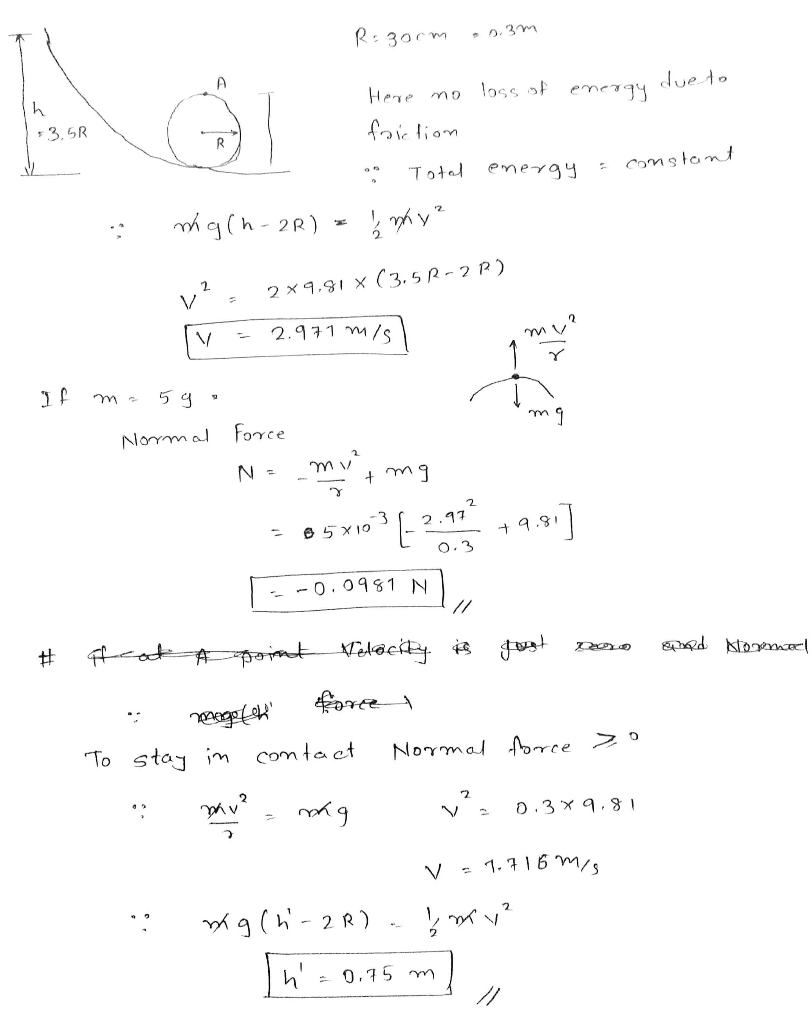#### Earn Coins

Coins can be redeemed for fabulous gifts.

Similar Homework Help Questions
• ### A bead slides without friction around a loop-the-loop. The bead is released from a height 27.5...

A bead slides without friction around a loop-the-loop. The bead is released from a height 27.5 m from the bottom of the loop- the-loop which has a radius 9 m. The acceleration of gravity is 9.8 m/s2 . A) How large is the normal force on it at point A if its mass is 6 g

• ### 2. A bead slides without friction around a loop-the loop. The bead is released from a...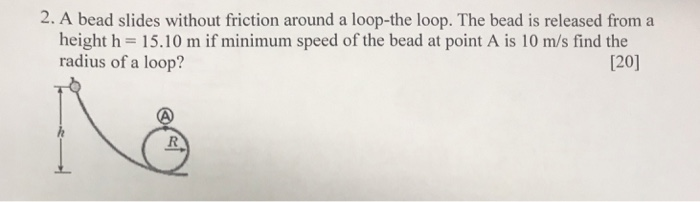2. A bead slides without friction around a loop-the loop. The bead is released from a height h 15.10 m if minimum speed of the bead at point A is 10 m/s find the radius of a loop? 120]

• ### A bead slides on a wire without friction around a loop-the-loop of radius 1.3 m.

A bead slides on a wire without friction around a loop-the-loop of radius 1.3 m. The bead is released with a speed 0.7 m/s from a height h=3.6 m where his measured from the bottom of the loop. How large is the normal force (in N) on the bead at the bottom of the loop, if its mass is 5 g?

• ### A bead slides on a wire without friction around a loop-the- loop of radius 1.1 m....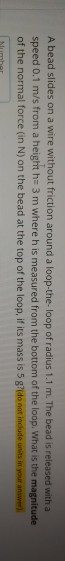A bead slides on a wire without friction around a loop-the- loop of radius 1.1 m. The bead is released with a speed 0.1 m/s from a height h 3 m whereh is measured from the bottom of the loop. What is the magnitude of the normal force (in N) on the bead at the top of the loop, if its mass is 5 gr(do nat include units in your answer)

• ### A bead with a hole through it slides on a wire track. The wire is threaded through the hole in the bead, and the bead slides without friction around a loop-the-loop (see figure below). The bead is released from rest at a height h = 3.90R.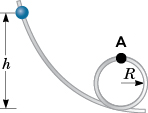A bead with a hole through it slides on a wire track. The wire is threaded through the hole in the bead, and the bead slides without friction around a loop-the-loop (see figure below). The bead is released from rest at a height h = 3.90R.

• ### A bead with a hole through it slides on a wire track. The wire is threaded through the hole in the bead, and the bead...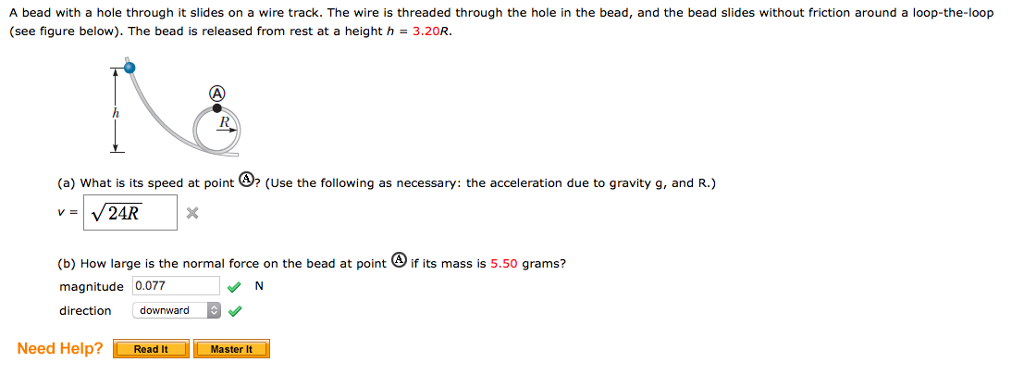A bead with a hole through it slides on a wire track. The wire is threaded through the hole in the bead, and the bead slides without friction around a loop-the-loop (see figure below). The bead is released from rest at a height h 3.20R (a) what is its speed at point (Use the following as necessary: the acceleration due to gravity g, and R.) (b) How large is the normal force on the bead at point if its mass...

• ### A bead with a hole through it slides on a wire track. The wire is threaded...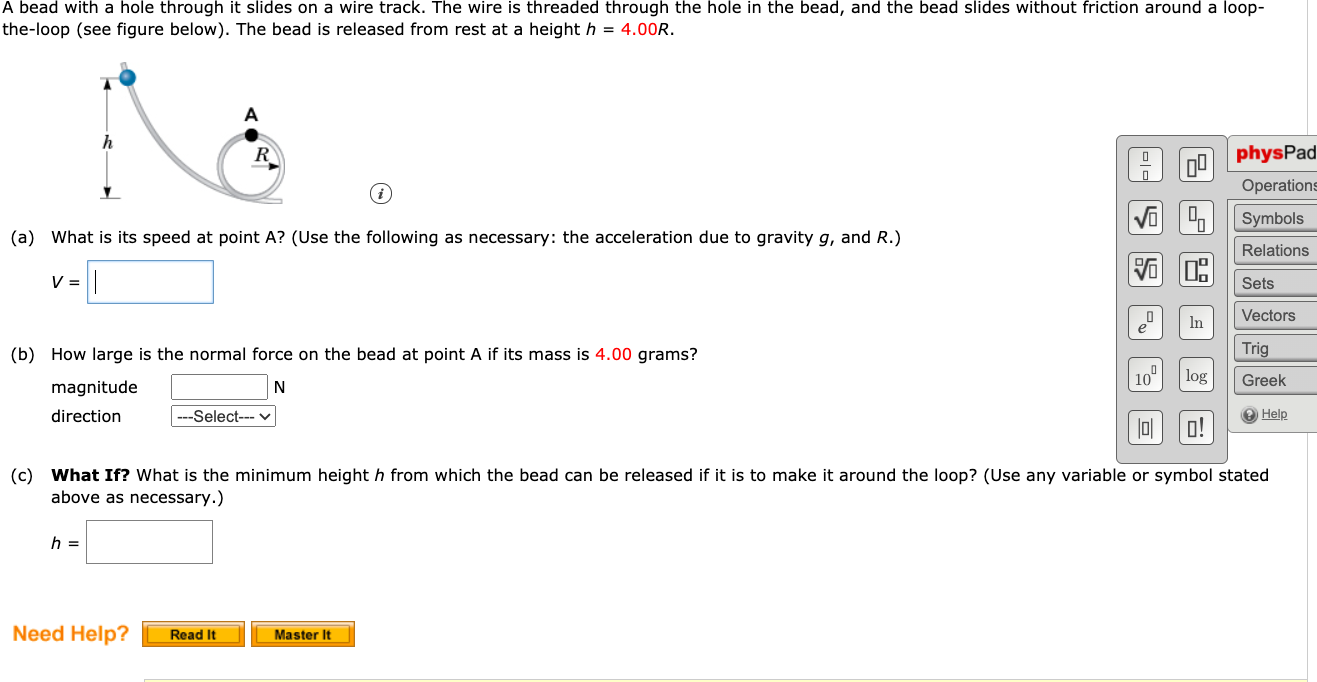A bead with a hole through it slides on a wire track. The wire is threaded through the hole in the bead, and the bead slides without friction around a loop- the-loop (see figure below). The bead is released from rest at a height h = 4.00R. h 00 physPad Operations Symbols VO (a) What is its speed at point A? (Use the following as necessary: the acceleration due to gravity g, and R.) Relations V = Sets Vectors In...

• ### Physics

A bead slides without friction around a loop–the–loop (see figure below). The bead is released from rest at a height h = 3.40R.(a) What is its speed at point ? (Use the following as necessary: the acceleration due to gravity g, and R.)v =(b) How large is the normal force on the bead at point if its mass is 4.60 grams?magnitude Ndirection

• ### Im not sure if the work i have done so far is correct. Can i have...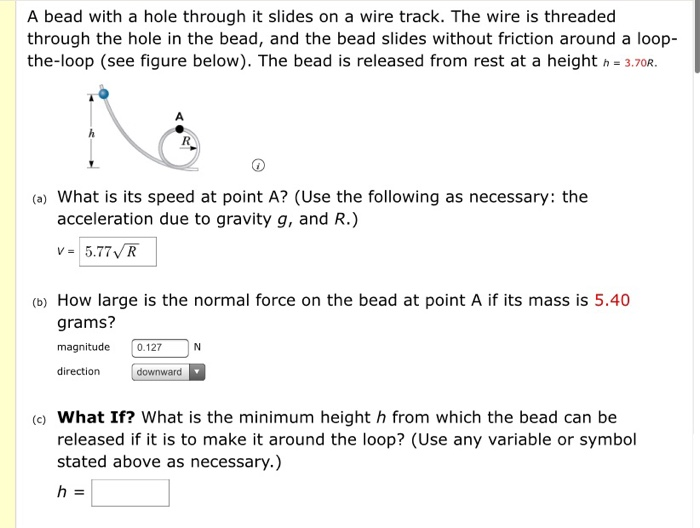Im not sure if the work i have done so far is correct. Can i have help with each part of this question? A bead with a hole through it slides on a wire track. The wire is threaded through the hole in the bead, and the bead slides without friction around a loop- the-loop (see figure below). The bead is released from rest at a height n = 3.70R. (a) What is its speed at point A? (Use the...